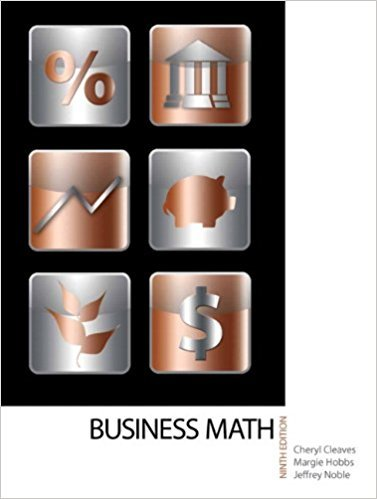×
×

# Solutions for Chapter 5-2: USING EQUATIONS TO SOLVE PROBLEMS## Full solutions for Business Math, | 9th Edition

ISBN: 9780135108178Solutions for Chapter 5-2: USING EQUATIONS TO SOLVE PROBLEMS

Solutions for Chapter 5-2
4 5 0 367 Reviews
21
3
##### ISBN: 9780135108178

This textbook survival guide was created for the textbook: Business Math, , edition: 9. Chapter 5-2: USING EQUATIONS TO SOLVE PROBLEMS includes 16 full step-by-step solutions. Since 16 problems in chapter 5-2: USING EQUATIONS TO SOLVE PROBLEMS have been answered, more than 19376 students have viewed full step-by-step solutions from this chapter. Business Math, was written by and is associated to the ISBN: 9780135108178. This expansive textbook survival guide covers the following chapters and their solutions.

Key Math Terms and definitions covered in this textbook
• Block matrix.

A matrix can be partitioned into matrix blocks, by cuts between rows and/or between columns. Block multiplication ofAB is allowed if the block shapes permit.

• Cofactor Cij.

Remove row i and column j; multiply the determinant by (-I)i + j •

• Companion matrix.

Put CI, ... ,Cn in row n and put n - 1 ones just above the main diagonal. Then det(A - AI) = ±(CI + c2A + C3A 2 + .•. + cnA n-l - An).

• Cross product u xv in R3:

Vector perpendicular to u and v, length Ilullllvlll sin el = area of parallelogram, u x v = "determinant" of [i j k; UI U2 U3; VI V2 V3].

• Dimension of vector space

dim(V) = number of vectors in any basis for V.

• Eigenvalue A and eigenvector x.

Ax = AX with x#-O so det(A - AI) = o.

• Fast Fourier Transform (FFT).

A factorization of the Fourier matrix Fn into e = log2 n matrices Si times a permutation. Each Si needs only nl2 multiplications, so Fnx and Fn-1c can be computed with ne/2 multiplications. Revolutionary.

• Full row rank r = m.

Independent rows, at least one solution to Ax = b, column space is all of Rm. Full rank means full column rank or full row rank.

• Hessenberg matrix H.

Triangular matrix with one extra nonzero adjacent diagonal.

• Iterative method.

A sequence of steps intended to approach the desired solution.

• Least squares solution X.

The vector x that minimizes the error lie 112 solves AT Ax = ATb. Then e = b - Ax is orthogonal to all columns of A.

• Orthogonal matrix Q.

Square matrix with orthonormal columns, so QT = Q-l. Preserves length and angles, IIQxll = IIxll and (QX)T(Qy) = xTy. AlllAI = 1, with orthogonal eigenvectors. Examples: Rotation, reflection, permutation.

• Rank one matrix A = uvT f=. O.

Column and row spaces = lines cu and cv.

• Rayleigh quotient q (x) = X T Ax I x T x for symmetric A: Amin < q (x) < Amax.

Those extremes are reached at the eigenvectors x for Amin(A) and Amax(A).

• Schur complement S, D - C A -} B.

Appears in block elimination on [~ g ].

• Similar matrices A and B.

Every B = M-I AM has the same eigenvalues as A.

• Singular Value Decomposition

(SVD) A = U:E VT = (orthogonal) ( diag)( orthogonal) First r columns of U and V are orthonormal bases of C (A) and C (AT), AVi = O'iUi with singular value O'i > O. Last columns are orthonormal bases of nullspaces.

• Skew-symmetric matrix K.

The transpose is -K, since Kij = -Kji. Eigenvalues are pure imaginary, eigenvectors are orthogonal, eKt is an orthogonal matrix.

• Tridiagonal matrix T: tij = 0 if Ii - j I > 1.

T- 1 has rank 1 above and below diagonal.

• Wavelets Wjk(t).

Stretch and shift the time axis to create Wjk(t) = woo(2j t - k).

×